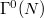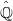﻿

### On the Action of$\Gamma ^0(N)$ on$\hat{\mathbb{Q}}$

#### Abstract

In this paper we examine$\Gamma ^0(N)$-orbits on$\hat{\mathbb{Q}}$and the suborbital graphs for$\Gamma ^0(N)$. Each such suborbitalgraph is a disjoint union of subgraphs whose vertices form a blockof imprimitivity for$\Gamma ^0(N)$. Moreover, these subgraphs areshown to be vertex$\Gamma ^0(N)$-transitive and edge$\Gamma^0(N)$-transitive. Finally, necessary and sufficient conditions forbeing self-paired edge are provided.

DOI Code: 10.1285/i15900932v30n2p141

Keywords: Congruence groups; Transitive and Imprimitive action; Suborbital graphs

Full Text: PDF